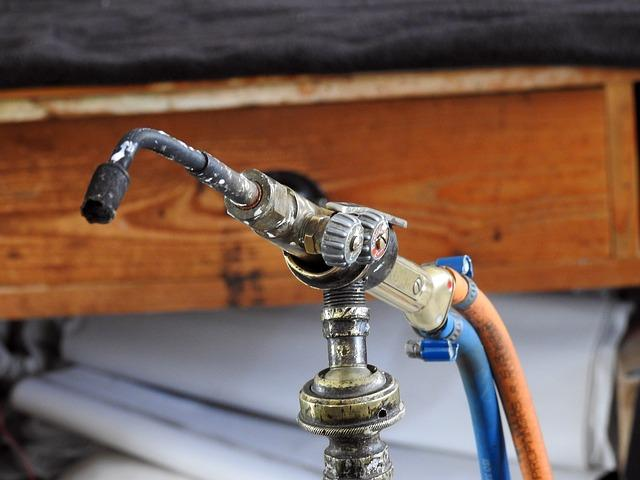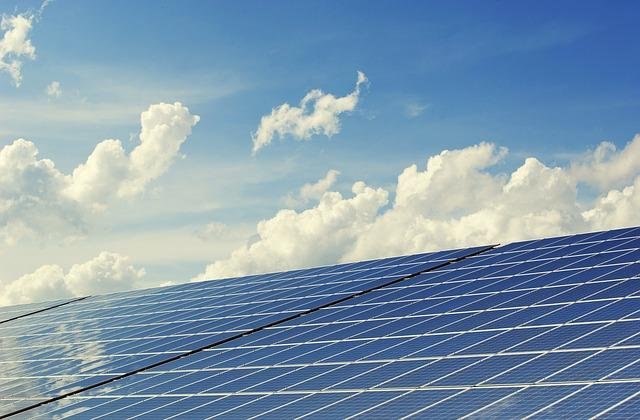Chapters

Many people don't opt for physics because they believe it is too difficult to prep. They assume physics lessons are all about long winding equations and derivatives. That is not the case. Physics is a scientific discipline that can be learned by whoever is interested in it.

Physics is the study of natural phenomena such as kinetics, waves, energy, work, power, and force.

High school students in the US sit for the SAT II physics exam in the last year of their high school. This helps them in gauging if they are ready for a college degree in the subject. The SAT subject test is used to ascertain if the student has an aptitude for the particular subject before taking it up as a career choice.

Superprof will explain the concepts of work, energy, and power in the physics SAT curriculum to help students decide if this subject is for them. A good supplementary syllabus to consider alongside the SAT physics syllabus is the physics syllabus for SAT prep.The best Physics tutors available
1st lesson free!5 (10 reviews)
Rao
\$15
/h
1st lesson free!5 (12 reviews)
Jaymin
\$15
/h
1st lesson free!5 (7 reviews)
Matthew
\$35
/h
1st lesson free!Delano
\$30
/h
1st lesson free!Santiago
\$10
/h
1st lesson free!5 (3 reviews)
Sergio
\$20
/h
1st lesson free!5 (5 reviews)
Austin
\$30
/h
1st lesson free!5 (4 reviews)
Marin
\$30
/h
1st lesson free!5 (10 reviews)
Rao
\$15
/h
1st lesson free!5 (12 reviews)
Jaymin
\$15
/h
1st lesson free!5 (7 reviews)
Matthew
\$35
/h
1st lesson free!Delano
\$30
/h
1st lesson free!Santiago
\$10
/h
1st lesson free!5 (3 reviews)
Sergio
\$20
/h
1st lesson free!5 (5 reviews)
Austin
\$30
/h
1st lesson free!5 (4 reviews)
Marin
\$30
/h

## Work

Work is a scalar quantity that is the product of the force applied on an object and the resulting displacement due to this force. Generally, work is done by some applied force.

When a force is applied to a body, causing it to move, it is said to do work. Work is said to be completed when energy has been transferred. In simple words, the energy transferred = work done.

The unit for measuring work is joules (J).

Calculating work is dependent on the quantity of force applied and the distance moved by the object in response. Therefore, the equation for work is w = f x d, where f is force, and d is the distance moved.

The measuring force units are Newtons (N), and distance is measured in meters (m). The distance must be in the same direction as the force applied.

When force and displacement are parallel, it is easy to calculate work. All you have to do is multiply the two quantities. However, if the two are not parallel, then things become slightly more complicated.

To calculate work in this scenario, the force's x and y components are taken into account.

If force and displacement are perpendicular to each other, then no work is done.

## Energy

Energy is an important part of physics, and it relates to work. Students will get to learn the definitions and real-life examples related to energy. They will also learn to use a few mathematical equations to solve work and energy-related physics problems

The basic principle of energy to keep in mind during your prep is that no new energy can be created or destroyed. It can only be converted from one form to another. We will take into account the different kinds of energy we deal with in nature.

• Magnetic - this energy comes into play when two like poles repel or two unlike poles attract. You can find common examples of this energy in fridge magnets and compasses
• Thermal - this kind of energy is stored in objects of high temperatures. The hotter the object, the more thermal energy it has
• Chemical - this energy is the energy stored inside chemical bonds, and it is found in exothermic and endothermic reactions
• Kinetic - this energy can be seen in moving cars, buses, and trains
• Electrostatic - this energy comes into play when like charges are moved closer together or attracting charges are moved away. This is observed in thunderclouds or static on clothes. Learn more about electricity
• Elastic potential - this kind of energy is stored when objects are stretched or squashed. This is observed in stretched rubber bands
• Gravitational potential - this energy is seen in objects that are at a height from the ground. Some examples are aeroplanes and kites.

Energy can stay preserved in an object for countless years, or just a millisecond. You can observe the transfer of energy happening around you all the time. There are four common ways energy is transferred, and these are:

1. Mechanical work
2. Electrical work
3. Heating

Note that work here is another way of saying energy transfer. The two terms are interchangeable.

Students sitting for their physics subject test are also taught the use of equations to calculate kinetic energy using an important equation.

## Power

The next subtopic students must prepare for their physics exam is power. Power is the rate of energy transfer per second. The equation for power is calculated as p=w/t, where w is work, and t is time.

The unit for power is watts (W), work done is taken in joules (J), and time in seconds (s).

For every joule transferred per second, the power increases by a value of a watt.The best Physics tutors available
1st lesson free!5 (10 reviews)
Rao
\$15
/h
1st lesson free!5 (12 reviews)
Jaymin
\$15
/h
1st lesson free!5 (7 reviews)
Matthew
\$35
/h
1st lesson free!Delano
\$30
/h
1st lesson free!Santiago
\$10
/h
1st lesson free!5 (3 reviews)
Sergio
\$20
/h
1st lesson free!5 (5 reviews)
Austin
\$30
/h
1st lesson free!5 (4 reviews)
Marin
\$30
/h
1st lesson free!5 (10 reviews)
Rao
\$15
/h
1st lesson free!5 (12 reviews)
Jaymin
\$15
/h
1st lesson free!5 (7 reviews)
Matthew
\$35
/h
1st lesson free!Delano
\$30
/h
1st lesson free!Santiago
\$10
/h
1st lesson free!5 (3 reviews)
Sergio
\$20
/h
1st lesson free!5 (5 reviews)
Austin
\$30
/h
1st lesson free!5 (4 reviews)
Marin
\$30
/h

## Efficiency

Devices are manufactured in a way that they waste the least amount of energy. The aim is to transfer as much energy as received into useful energy output. Efficiency is a measure of how perfectly a device converts the energy input into an output.

The equation for efficiency is the amount of useful energy divided by total energy supplied.

## Types Of Energy

There are different forms in which energy is stored around us. Some examples of energy stores taught in physics lessons are:

### Gravitational Potential Energy

An object stores energy due to its position. An object at height will have more stored energy than one on the ground. Gravitational potential energy is the energy stored in an object as a result of its height. It depends on the mass of the object plus the height it is at.

### Elastic Potential Energy

This is the type of energy stored in an object as a result of its stretching or compressing. Elastic potential energy can be stored in bungee cords, a stretched rubber band, springs, etc.

To measure the elastic potential energy in the spring, we use Hooke's law, where f is equal to the product of k into x. It states that the amount of force is directly proportional to the amount of stretch (x) and the spring constant (k).Kinetic Energy

An object in motion possesses energy, which is known as kinetic energy. It does not matter whether the motion is vertical or horizontal.  The types of kinetic energy can vary, such as rotational kinetic energy, vibrational kinetic energy, and translational kinetic energy.

Generally, the most common form is translational kinetic energy. It is calculated as the product of half the mass of the object with its velocity squared.

### Thermal Energy

Thermal energy refers to the energy within a system of thermodynamic equilibrium due to its temperature. There is a whole branch of physics called thermodynamics that deals with how heat is transferred between systems.

### Magnetic Energy

Magnetic energy is the amount of energy stored inside a magnetic field. All permanent magnets have stored magnetic energy within them.

The energy within a magnetic field is the energy required to align the magnets within the system in a parallel arrangement. To learn more about this phenomenon, check out the topic of magnetism and electromagnetism.

### Conservation Of Energy

It is a known fact that energy cannot be created or destroyed. It can only be converted from one form to another, such as when you burn fuel (thermal energy) to run your car (kinetic energy).

This means that a closed system will always have the same amount of energy. The amount of energy in a system is determined by the system's total internal energy, the initial internal energy plus the work done on the system, and any heat added or removed to the system.

### Energy And Heating

In their next physics lessons, students learn about the basics of thermal energy. Energy is transmitted in three ways, that is, conduction, convection, and radiation.

Conduction is the transfer of energy inside a solid. Metals are very good conductors of heat, while wood is not. You will understand the phenomenon of conduction better when you study the particle model of matter.

Convection is the transfer of energy inside a fluid. It depends upon density. Air and water are both fluids, but air does not transfer heat well, while water does. Convection is a slower process than conduction.

Radiation is the process of transmitting energy through electromagnetic waves. It does not require any medium. The heat we get from the sun reaches us through radiation.

The conductivity of a material can be gauged by evaluating the time taken to transfer energy through them.

Materials that allow internal energy to be transmitted easily are conductors. All metals are good conductors.

Insulators are materials that hinder the transfer of internal energy. A good example of insulators is wood and rubber.Bunsen burners can be used to used in scientific experiments to determine thermal conductivity. (Source: pixabay)

## Thermal Conductivity

Many experiments can be done to determine the efficiency of materials transferring energy when heated.

Thermal conductivity is measured in watts per meter per degree Celsius.

Students learn to test different ways of insulation. This is done by noting the time they take to heat up and the temperature they reach. Here are some links to understand the methods; part one and part two.

## Energy Demands

All living things participate in the transfer of energy. The energy we transfer in our daily lives is supplied by electricity generated from different energy sources.

There are different types of energy sources in the world. They can be both renewable and non-renewable.

These energy resources can be fossil fuels, biofuels, hydroelectric power, and nuclear power.

Renewable sources can be regenerated and rely on the forces of nature, such as tidal power, hydroelectric power, solar power, nuclear power, etc. The topic of atomic structure and nuclear energy are closely related to each other.

Non-renewable sources of energy are stored inside the earth in the form of hydrocarbons and fuels. These are finite and will run out eventually. A few examples of non-renewable sources of energy are natural gas and oil.

Some of the places which incorporate energy are homes, offices, public transport, and industrial plants.

It's a good idea to go through other unique topics of the physics syllabus, such as space physics.Energy from the sun or solar power is a renewable energy that is commonly used today. (Source: pixabay)

Sign up now with Superprof and choose from a range of qualified SAT II physics tutors who are experts in their field.5.00/5 - 1 vote(s)Loading...